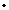Program
ORGANIZERS
V Balaji (CMI, India), I Biswas (TIFR, India) and A Parameswaran (TIFR, India)
DATE & TIME
21 March 2016 to 01 April 2016
VENUE

Higgs bundles arise as solutions to noncompact analog of the Yang-Mills equation. Hitchin showed that irreducible solutions of the GL(2,C) Yang-Mills equation on a compact Riemann surface X are precisely the polystable Higgs vector bundles on X of rank two and degree zero. Subsequently Simpson proved that irreducible solutions of the GL(r,C) Yang-Mills equation on a compact Kaehler manifold X are precisely the polystable Higgs vector bundles on X of rank r  and vanishing Chern classes.

Hitchin showed that the moduli spaces of stable Higgs bundles give examples of hyper-Kaehler manifolds and provide examples of completely integrable systems. Simpson proved basic theorems on fundamental group of Kaehler manifolds using the identification of Higgs bundles with the solutions of the Yang-Mills equation.

The moduli space of Higgs bundles is then full of rich geometric structures, but the most interesting part of the story is not just this but the different points of view that one can use for studying this moduli space, which show this object as the precise mathematical context for different physical theories. For instance, the moduli space of SL(2, C)-Higgs bundles was proved by T. Hausel and M. Thaddeus, to be the first non-trivial example for Strominger-Yau-Zaslow formulation of Mirror symmetry (symplectic formulation concerning pairs of Calabi-Yau manifolds) satisfying at the same time the Batyrev-Borisov mirror symmetry definition (which is comprised of a condition on the Hodge numbers of those manifolds).

Higgs bundles play a very central role in the works of G. Laumon, L. Lafforgue and B.C. Ngô in their work on Langlands program (the last two were awarded Field's medal in 2002 and 2010 respectively). The Geometric Langlands Program, in terms of the Monotonen-Olive duality conjecture, states that maximally supersymmetric gauge theory in four dimensions with gauge group G is isomorphic to a similar gauge theory with gauge group being the Langlands dual of G. From the physical viewpoint, the Monotonen-Olive duality can be regarded as a nonabelian  generalization of electric magnetic duality. Gukov and Witten interpreted the ramified (or punctured) version of the Geometric Langlands Program in physical terms.

There will be two short courses during the program:

1. Tomas L. Gomez will lecture on the work of Hitchin-Donaldson-Corlette-Simpson which prove that solutions of the Yang-Mills-Higgs equation are precisely the polystable Higgs bundles with vanishing Chern classes.
2. Laura Schaposnik will lecture on the completely integrable systems given by the moduli spaces of Higgs bundles on a Riemann surface.

Some participants will deliver one lecture each and there will be about 30 talks.

higgsbundlesictsresin